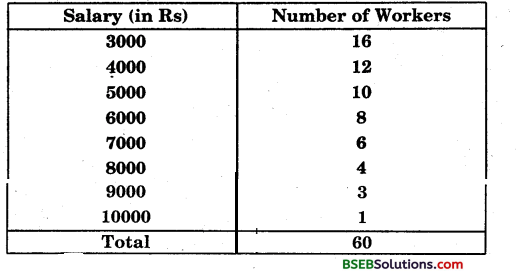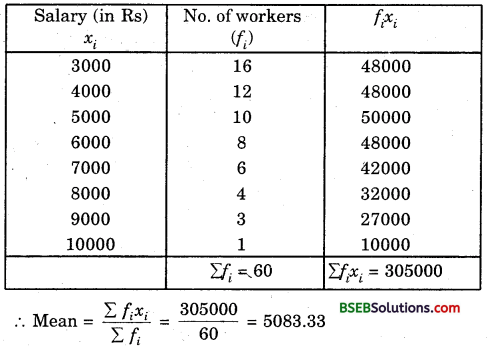# Bihar Board Class 9th Maths Solutions Chapter 14 Statistics Ex 14.4

Bihar Board Class 9th Maths Solutions Chapter 14 Statistics Ex 14.4 Textbook Questions and Answers.

## BSEB Bihar Board Class 9th Maths Solutions Chapter 14 Statistics Ex 14.4Question 1.
The following number of goals were scored by a team in a series of 10 matches:
2, 3, 4, 5, 0, 1, 3, 3, 4, 3.
Find the mean, median and mode of these scores.
Solution:
Using $$\bar{x}$$ = $$\frac {x_1+x_2+…….+x_{10}}{10}$$, the mean is
$$\bar{x}$$ = $$\frac {2 + 3 + 4 + 5 + 04-1 + 3 + 3 + 4 + 3}{10}$$
= $$\frac {28}{10}$$ = 2.8
To find the median, arrange the given data in ascending order, as follows
0, 1, 2, 3, 3, 3, 3, 4, 4, 5.
There are 10 terms. So, there are two middle terms, i.e.,
the ($$\frac {10}{2}$$)th and ($$\frac {10}{2}$$ + 1)th
i.e., the 5th and 6th terms.
So, the median is the mean of the values of the 5th and 6th terms.
i.e., the median = $$\frac {3+3}{2}$$ = 3
Again, in the data 3 occurs most frequently i.e., 4 times. So, mode = 3.Question 2.
In a Mathematics test given to 15 students, the following marks (out of 100) are recorded:
41, 39, 48, 52, 46, 62, 54, 40, 96, 52, 98, 40, 42, 52, 60
Find the mean, median and mode of this data.
Solution:
Using $$\bar{x}$$ = $$\frac {x_1+x_2+…….+x_{15}}{10}$$, the mean is
$$\bar{x}$$ = $$\frac {41 + 39 + 48 + 52 + 46 + 62 + 54 + 40 + 96 + 52 + 98 + 40 + 42 + 52 + 60}{15}$$
= $$\frac {822}{15}$$ = 54.8
To find the median, arrange the given data in ascending order, as follows:
39, 40, 40, 41, 42, 46, 48, 52, 52, 52, 54, 60, 62, 96, 98.
Since the number of terms is 15, an odd number, we find out the median by finding the marks obtained by ($$\frac {15+1}{2}$$)th student, which is 8th student.
∴ The median marks = 52
Again, in the data 52 occurs most frequently i.e., 3 times.
∴ Mode = 52.Question 3.
The following observations have been arranged in ascending order. If the median of the data is 63, find the value of x.
29, 32, 48, 50, x, x + 2, 72, 78, 84, 95.
Solution:
The given arranged in ascending order is as
29, 32, 48, 50, x, x + 2, 72, 78, 84, 95
There are 10 terms. So, there are two middle terms, i.e.,
the ($$\frac {10}{2}$$)th and ($$\frac {10}{2}$$ + 1)th i.e., the 5th and 6th terms.
So, the median is the mean of the values of the 5th and 6 th terms.
i.e., the median = $$\frac {x + (x + 2)}{2}$$ = x + 1
But median = 63
Given
∴ x + 1 = 63
⇒ x = 63 – 1 = 62.Question 4.
Find the mode
14,25, 14, 28, 18, 17, 18, 14, 23, 22, 14, 18
Solution:
Arranging the given data in ascending order as follows, we get
14, 14, 14, 14, 17, 18, 18, 18, 22, 23, 25, 28.
Here 14 occurs most frequently i.e., 4 times.
∴ Mode = 14.Question 5.
Find the mean salary of 60 workers of a factory from the following table:Solution:
Calculation of mean:Thus, mean salary of 60 workers is Rs 5083.33.Question 6.
Give one example of a situation in which
(i) the mean is an appropriate measure of central tendency.
(ii) the mean is not an appropriate measure of central tendency but the median is an appropriate measure of central tendency.
Solution:
(i) The mean is an appropriate measure because of its unique value and can be used to compare different groups of data.
(ii) For the measurement of qualitative characteristics e.g., beauty, honesty, intelligence etc., mean cannot be used.